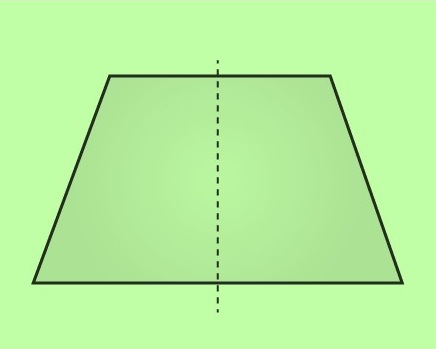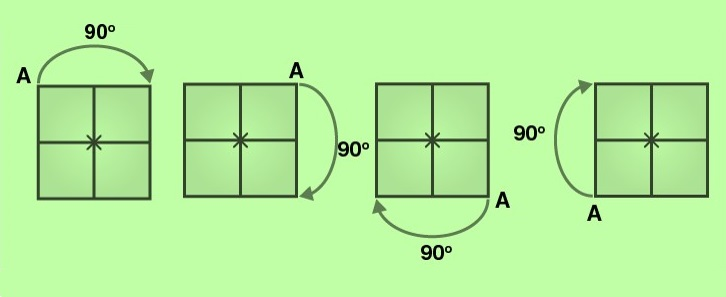# vs.eyeandcontacts.com

## Chapter 14  Symmetry Exercise 14.3

Question 1: Name any two figures that have both line symmetry and rotational symmetry.
Equilateral triangle and Circle are the 2 figures that have both line symmetry and rotational symmetry.

Question 2: Draw, wherever possible, a rough sketch of
(i) A triangle with both line and rotational symmetries of order more than 1.

Line symmetryRotational symmetry(ii) A triangle with only line symmetry and no rotational symmetry of order more than 1.(iii) A quadrilateral with a rotational symmetry of order more than 1 but not a line symmetry.
A quadrilateral with a rotational symmetry of order more than 1 but not a line symmetry cannot be drawn as a quadrilateral with a line symmetry may have rotational symmetry of order one but not more than one.

(iv) A quadrilateral with line symmetry but not a rotational symmetry of order more than 1.Question 3: If a figure has two or more lines of symmetry, should it have rotational symmetry of order more than 1?
Yes, if a figure has two or more lines of symmetry, then it will have rotational symmetry of order more than 1.

Question 4: Fill in the blanks:

 Shape Centre of Rotation Order of Rotation Angle of Rotation Square Rectangle Rhombus Equilateral Triangle Regular Hexagon Circle Semi-circle

 Shape Centre of Rotation Order of Rotation Angle of Rotation Square Intersecting point of diagonals 4 90o Rectangle Intersecting point of diagonals 2 180o Rhombus Intersecting point of diagonals 2 180o Equilateral Triangle Intersecting point of medians 3 120o Regular Hexagon Intersecting point of diagonals 6 60o Circle Centre Infinite Every angle Semi-circle Mid-point of diameter 1 360o

Question 5: Name the quadrilaterals which have both line and rotational symmetry of order more than 1.
The quadrilateral which have both line and rotational symmetry of order more than 1 is a square.
Line symmetry:Rotational symmetry:Question 6: After rotating by 60° about a centre, a figure looks exactly the same as its original position. At what other angles will this happen for the figure?
The other angles are 120°, 180°, 240°, 300°, and 360°.

Question 7: Can we have a rotational symmetry of order more than 1 whose angle of rotation is
(i) 45°?